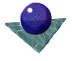Solving for
Perspective InversionWe assume that we have identified a group of points in the image, probably junctions between straight lines, but possibly other control points such as ellipse centres which grouping suggests may correspond to similar model control points. Now we have to solve the perspective-n-point problem; i.e. given a set of n corresponding points what is the pose of the object in 3D space ? Usually n is 3 or 4. ( Less than this gives an infinite number of solutions.) When n=3 there are a maximum of eight possible solutions, of which only four occur in real 3D space in front of the camera. If n=4 and these points are coplanar, there is a unique solution; otherwise for non-coplanar points there are two solutions. We shall deal only with the case of 3 points, illustrated in Figure 6, below. We refer mainly to the article by Fischler and Bolles [Reference no 1] although this problem has been discussed by many authors.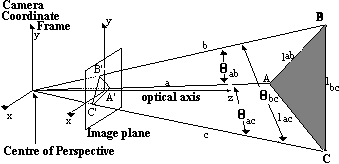Figure 6:Solving the perspective-3-point problem

The basic problem is to determine the lencths of the three legs of the tetrahedron formed by A,B,C and the centre of perspective CP. From trigonometry, we can form three equations based on the Cosine rule,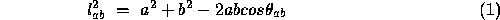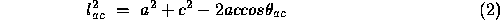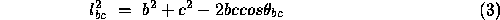Making the substitutions

i.e. expressing the distances to points B and C as a constant times the distance to point A, we obtain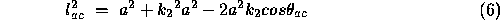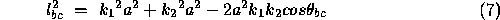It is possible to combine and re-arrange equations 5,6 and 7 to form a quartic equation in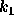.

where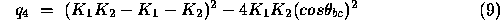(note the G3 in the following corrected equation should be q3):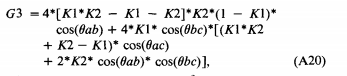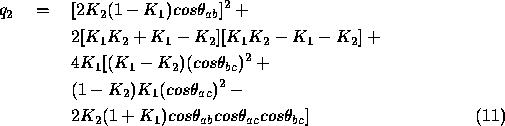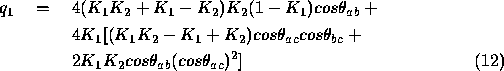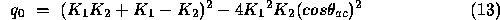and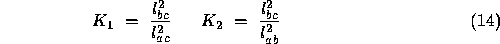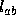,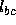and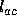are known from the rigid model of the object, since we have assumed point correspondence. For each positive real root of the Equation 8 we can then calculate the values of a,b and c, i.e. substituting for k1 in Equation 5, below. This is simply a rearrangement of Equation 5 Then b and c are known directly from Equations 16 and 17 ( i.e. Equation 4). To determine c, we also need to calculate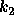. There are two possible conditions, given in Equations 18 and 19.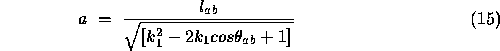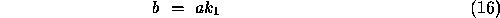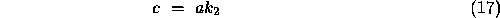where, either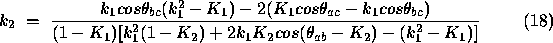provided the denominator is not equal to zero, or, if this test fails then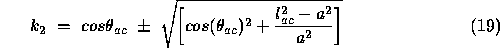As stated this system of equations has a maximum of eight solutions [See Reference no 1], but four of these solutions have negative values of a,b and c - they are behind the camera and are isomorphic to the other four solutions which are in the world in front of the camera. Figure 7 gives pictorial example of how these multiple solutions may occur.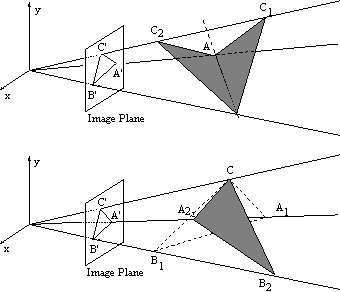Figure 7:   Multiple solutions to the perspective-3-point problemComments to: Sarah Price at ICBL.